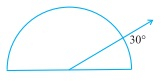# InvestigateIn the given figure, the angle measures $30^{\circ}$. Look at the same figure through a magnifying glass. Does the angle becomes larger? Does the size of the angle change?"

To do:

We have to find whether the angle becomes larger when seen through a magnifying glass.

Solution:

The measure of the angle remains $30^o$ even when we look at it through a magnifying glass.

Therefore,

The measure of an angle does not change by viewing through a magnifying glass.